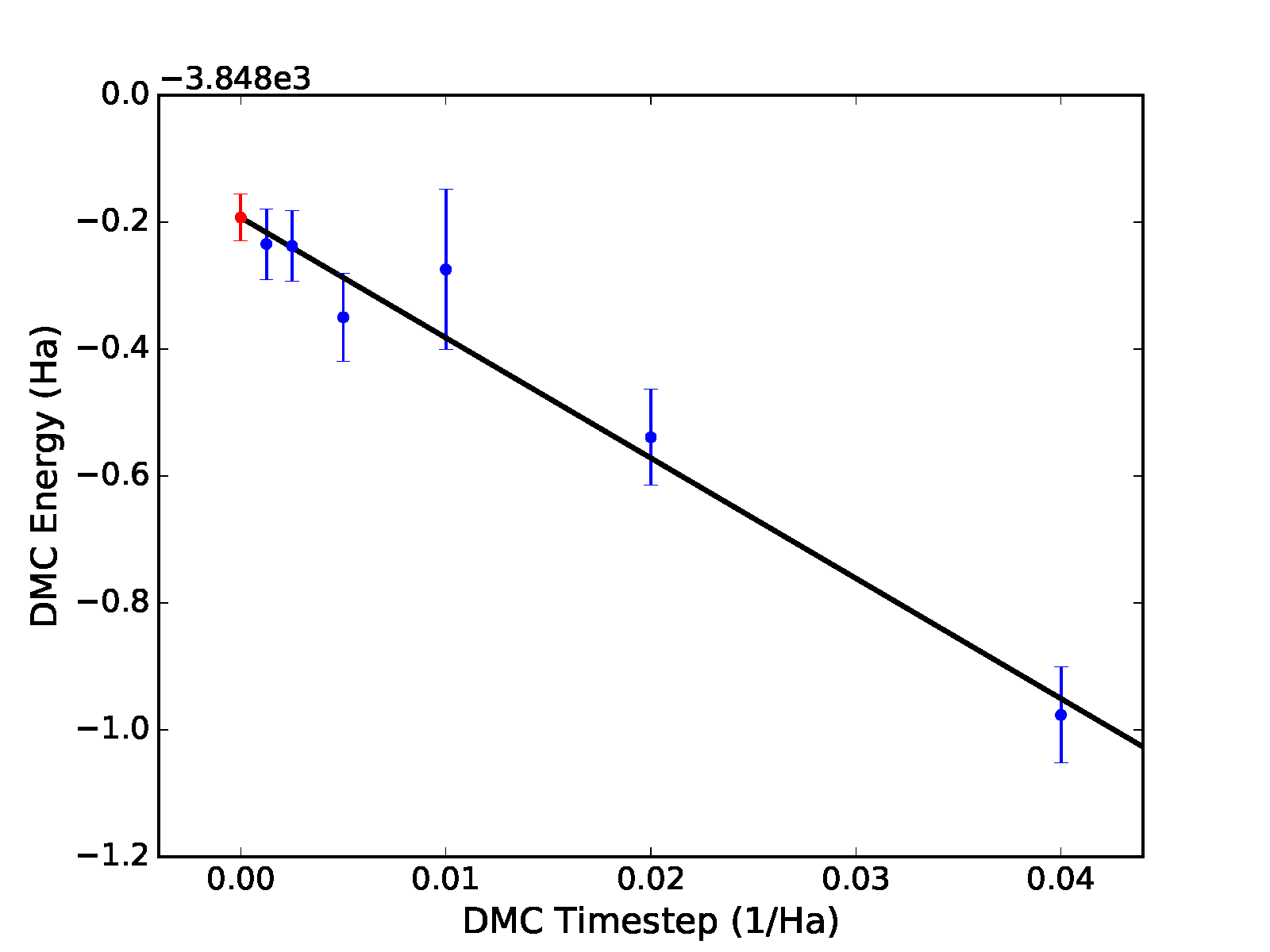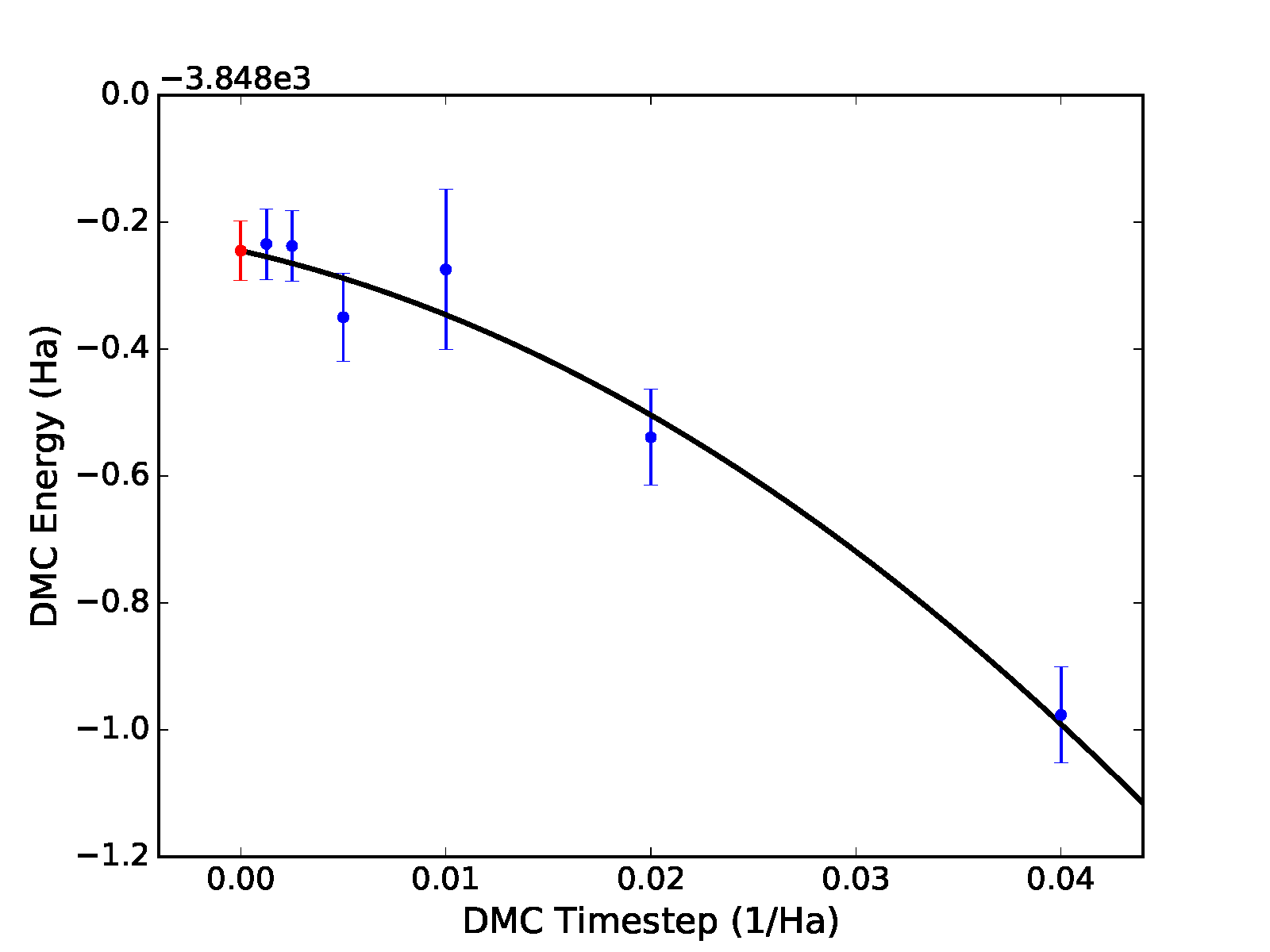# Analyzing QMCPACK data¶

## Using the qmc-fit tool for statistical time step extrapolation and curve fitting¶

The qmc-fit tool is used to provide statistical estimates of curve-fitting parameters based on QMCPACK data. Although qmc-fit will eventually support many types of fitted curves (e.g., Morse potential binding curves and various equation-of-state fitting curves), it is currently limited to estimating fitting parameters related to time step extrapolation.

### The jackknife statistical technique¶

The qmc-fit tool obtains estimates of fitting parameter means and associated error bars via the “jack-knife” technique. This technique is a powerful and general tool to obtain meaningful error bars for any quantity that is related in a nonlinear fashion to an underlying set of statistical data. For this reason, we give a brief overview of the jackknife technique before proceeding with usage instructions for the qmc-fit tool.

Consider $$N$$ statistical variables $$\{x_n\}_{n=1}^N$$ that have been outputted by one or more simulation runs. If we have $$M$$ samples of each of the $$N$$ variables, then the mean values of each these variables can be estimated in the standard way, that is, $$\bar{x}_n\approx \tfrac{1}{M}\sum_{m=1}^Mx_{nm}$$.

Suppose we are interested in $$P$$ statistical quantities $$\{y_p\}_{p=1}^P$$ that are related to the original $$N$$ variables by a known multidimensional function $$F$$:

(45)\begin{split}\begin{aligned} y_1,y_2,\ldots,y_P &= F(x_1,x_2,\ldots,x_N)\quad \textrm{or} \nonumber \\ \vec{y} &= F(\vec{x})\:.\end{aligned}\end{split}

The relationship implied by $$F$$ is completely general. For example, the $$\{x_n\}$$ might be elements of a matrix with $$\{y_p\}$$ being the eigenvalues, or $$F$$ might be a fitting procedure for $$N$$ energies at different time steps with $$P$$ fitting parameters. An approximate guess at the mean value of $$\vec{y}$$ can be obtained by evaluating $$F$$ at the mean value of $$\vec{x}$$ (i.e. $$F(\bar{x}_1\ldots\bar{x}_N)$$), but with this approach we have no way to estimate the statistical error bar of any $$\bar{y}_p$$.

In the jackknife procedure, the statistical variability intrinsic to the underlying data $$\{x_n\}$$ is used to obtain estimates of the mean and error bar of $$\{y_p\}$$. We first construct a new set of $$x$$ statistical data by taking the average over all samples but one:

(46)$\tilde{x}_{nm} = \frac{1}{N-1}(N\bar{x}_n-x_{nm})\qquad m\in [1,M]\:.$

The result is a distribution of approximate $$x$$ mean values. These are used to construct a distribution of approximate means for $$y$$:

(47)$\tilde{y}_{1m},\ldots,\tilde{y}_{Pm} = F(\tilde{x}_{1m},\ldots,\tilde{x}_{Nm}) \qquad m\in [1,M]\:.$

Estimates for the mean and error bar of the quantities of interest can finally be obtained using the following formulas:

(48)\begin{split}\begin{aligned} \bar{y}_p &= \frac{1}{M}\sum_{m=1}^M\tilde{y}_{pm}\:. \\ \sigma_{y_p} &= \sqrt{\frac{M-1}{M}\left(\sum_{m=1}^M\tilde{y}_{pm}^2-M\bar{y}_p^2\right)}\:.\end{aligned}\end{split}

### Performing time step extrapolation¶

In this section, we use a 32-atom supercell of MnO as an example system for time step extrapolation. Data for this system has been collected in DMC using the following sequence of time steps: $$0.04,~0.02,~0.01,~0.005,~0.0025,~0.00125$$ Ha$$^{-1}$$. For a typical production pseudopotential study, time steps in the range of $$0.02-0.002$$ Ha$$^{-1}$$ are usually sufficient and it is recommended to increase the number of steps/blocks by a factor of two when the time step is halved. To perform accurate statistical fitting, we must first understand the equilibration and autocorrelation properties of the inputted local energy data. After plotting the local energy traces (qmca -t -q e -e 0 ./qmc*/*scalar*), it is clear that an equilibration period of $$30$$ blocks is reasonable. Approximate autocorrelation lengths are also obtained with qmca:

>qmca -e 30 -q e --sac ./qmc*/qmc.g000.s002.scalar.dat
./qmc_tm_0.00125/qmc.g000 series 2 LocalEnergy = -3848.234513 +/- 0.055754  1.7
./qmc_tm_0.00250/qmc.g000 series 2 LocalEnergy = -3848.237614 +/- 0.055432  2.2
./qmc_tm_0.00500/qmc.g000 series 2 LocalEnergy = -3848.349741 +/- 0.069729  2.8
./qmc_tm_0.01000/qmc.g000 series 2 LocalEnergy = -3848.274596 +/- 0.126407  3.9
./qmc_tm_0.02000/qmc.g000 series 2 LocalEnergy = -3848.539017 +/- 0.075740  2.4
./qmc_tm_0.04000/qmc.g000 series 2 LocalEnergy = -3848.976424 +/- 0.075305  1.8


The autocorrelation must be removed from the data before jackknifing, so we will reblock the data by a factor of 4.

The qmc-fit tool can be used in the following way to obtain a linear time step fit of the data:

>qmc-fit ts -e 30 -b 4 -s 2 -t '0.00125 0.0025 0.005 0.01 0.02 0.04' ./qmc*/*scalar*
fit function  : linear
fitted formula: (-3848.193 +/- 0.037) + (-18.95 +/- 1.95)*t
intercept     : -3848.193 +/- 0.037  Ha


The input arguments are as follows: ts indicates we are performing a time step fit, -e 30 is the equilibration period removed from each set of scalar data, -b 4 indicates the data will be reblocked by a factor of 4 (e.g., a file containing 400 entries will be block averaged into a new set of 100 before jackknife fitting), -s 2 indicates that the time step data begins with series 2 (scalar files matching *s000* or *s001* are to be excluded), and -t ‘0.00125 0.0025 0.005 0.01 0.02 0.04’ provides a list of time step values corresponding to the inputted scalar files. The -e and -b options can receive a list of file-specific values (same format as -t) if desired. As can be seen from the text output, the parameters for the linear fit are printed with error bars obtained with jackknife resampling and the zero time step “intercept” is $$-3848.19(4)$$ Ha. In addition to text output, the previous command will result in a plot of the fit with the zero time step value shown as a red dot, as shown in the top panel of Fig. 12.

Different fitting functions are supported via the -f option. Currently supported options include linear ($$a+bt$$), quadratic ($$a+bt+ct^2$$), and sqrt ($$a+b\sqrt{t}+ct$$). Results for a quadratic fit are shown subsequently and in the bottom panel of Fig. 12.

>qmc-fit ts -f quadratic -e30 -b4 -s2 -t '0.00125 0.0025 0.005 0.01 0.02 0.04' ./qmc*/*scalar*
fit function  : quadratic
fitted formula: (-3848.245 +/- 0.047) + (-7.25 +/- 8.33)*t + (-285.00 +/- 202.39)*t^2
intercept     : -3848.245 +/- 0.047  Ha


In this case, we find a zero time step estimate of $$-3848.25(5)$$ Ha$$^{-1}$$. A time step of $$0.04$$ Ha$$^{-1}$$ might be on the large side to include in time step extrapolation, and it is likely to have an outsize influence in the case of linear extrapolation. Upon excluding this point, linear extrapolation yields a zero timestep value of $$-3848.22(4)$$ Ha$$^{-1}$$. Note that quadratic extrapolation can result in intrinsically larger uncertainty in the extrapolated value. For example, when the $$0.04$$ Ha$$^{-1}$$ point is excluded, the uncertainty grows by 50% and we obtain an estimated value of $$-3848.28(7)$$ instead.Fig. 12 Linear (top) and quadratic (bottom) time step fits to DMC data for a 32-atom supercell of MnO obtained with qmc-fit. Zero time step estimates are indicated by the red data point on the left side of either panel.¶

## Using the qdens tool to obtain electron densities¶

The qdens tool is provided to post-process the heavy density data produced by QMCPACK and output the mean density (with and without errorbars) in file formats viewable with, e.g., XCrysDen or VESTA. The tool currently works only with the SpinDensity estimator in QMCPACK.

Note: this tool is provisional and may be changed or replaced at any time. The planned successor to this tool (qstat) will expand access to other observables and will retain at least the non-plotting capabilities of qdens.

To use qdens, Nexus must be installed along with NumPy and H5Py. A short list of example use cases are covered in the next section. Current input flags are:

>qdens

Usage: qdens [options] [file(s)]

Options:
--version             show program's version number and exit
-h, --help            Print help information and exit (default=False).
-v, --verbose         Print detailed information (default=False).
-f FORMATS, --formats=FORMATS
Format or list of formats for density file output.
Options: dat, xsf, chgcar (default=None).
-e EQUILIBRATION, --equilibration=EQUILIBRATION
Equilibration length in blocks (default=0).
-r REBLOCK, --reblock=REBLOCK
Block coarsening factor; use estimated autocorrelation
length (default=None).
-a, --average         Average over files in each series (default=False).
-w WEIGHTS, --weights=WEIGHTS
List of weights for averaging (default=None).
-i INPUT, --input=INPUT
QMCPACK input file containing structure and grid
information (default=None).
-s STRUCTURE, --structure=STRUCTURE
File containing atomic structure (default=None).
-g GRID, --grid=GRID  Density grid dimensions (default=None).
-c CELL, --cell=CELL  Simulation cell axes (default=None).
--lineplot=LINEPLOT   Produce a line plot along the selected dimension: 0,
1, or 2 (default=None).
--noplot              Do not show plots interactively (default=False).


### Usage examples¶

Process a single file, excluding the first 40 blocks, and produce XSF files:

qdens -v -e 40 -f xsf -i qmc.in.xml qmc.s000.stat.h5


Process files for all available series:

qdens -v -e 40 -f xsf -i qmc.in.xml *stat.h5


Combine groups of 10 adjacent statistical blocks together (appropriate if the estimated autocorrelation time is about 10 blocks):

qdens -v -e 40 -r 10 -f xsf -i qmc.in.xml qmc.s000.stat.h5


Apply different equilibration lengths and reblocking factors to each series (below is appropriate if there are three series, e.g. s000, s001, and s002):

qdens -v -e '20 20 40' -r '4 4 8' -f xsf -i qmc.in.xml *stat.h5


Produce twist averaged densities (also works with multiple series and reblocking):

qdens -v -a -e 40 -f xsf -i qmc.g000.twistnum_0.in.xml qmc.g*.s000.stat.h5


Twist averaging with arbitrary weights can be performed via the -w option in a fashion identical to qmca.

### Files produced¶

Look for files with names and extensions similar to:

qmc.s000.SpinDensity_u.xsf
qmc.s000.SpinDensity_u-err.xsf
qmc.s000.SpinDensity_u+err.xsf

qmc.s000.SpinDensity_d.xsf
qmc.s000.SpinDensity_d-err.xsf
qmc.s000.SpinDensity_d+err.xsf

qmc.s000.SpinDensity_u+d.xsf
qmc.s000.SpinDensity_u+d-err.xsf
qmc.s000.SpinDensity_u+d+err.xsf

qmc.s000.SpinDensity_u-d.xsf
qmc.s000.SpinDensity_u-d-err.xsf
qmc.s000.SpinDensity_u-d+err.xsf


Files postfixed with u relate to the up electron density, d to down, u+d to the total charge density, and u-d to the difference between up and down electron densities.

Files without err in the name contain only the mean, whereas files with +err/-err in the name contain the mean plus/minus the estimated error bar. Please use caution in interpreting the error bars as their accuracy depends crucially on a correct estimation of the autocorrelation time by the user (see -r option) and having a sufficient number of blocks remaining following any reblocking.

When twist averaging, the group tag (e.g. g000 or similar) will be replaced with avg in the names of the outputted files.